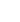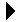# ConditionsCS 231 Lecture ExamplesDownload```; ; Tell if the three arguments are strictly sequenced. (defun inorder (a b c) (if (>= a b) () (if (>= b c) () t ) ) ) ; ; Tell if the three arguments are strictly sequenced. (defun inorder2 (a b c) (if (and (< a b) (< b c)) t nil ) ) ; ; Tell if the three arguments are strictly sequenced. (defun inorder3 (a b c) (and (< a b) (< b c))) ; ; Tell if the three arguments are strictly sequenced. (defun inorder4 (a b c) (cond ((>= a b) ()) ((>= b c) nil) (t t) ) ) ```